July 31, 2023

03 Hello Mesh

This example will focus on Mesh shaders and Hierarchical LOD graphs, also known as Nanite, within Tellusim Core SDK. Our goal is to draw 8192 mesh instances, each made from 90K triangles. A naive rendering of this scene will draw overwhelming 737M triangles, so we need LODs. Tellusim Core SDK has a lot of useful tools for mesh processing. The 90K triangles mesh is a subdivision surface generated from a simple 178 quads geometry. With the help of Crease attributes, we can precisely control the subdivision steps. Four consecutive subdivisions will result in the Mesh we need, exactly made from 90112 triangles: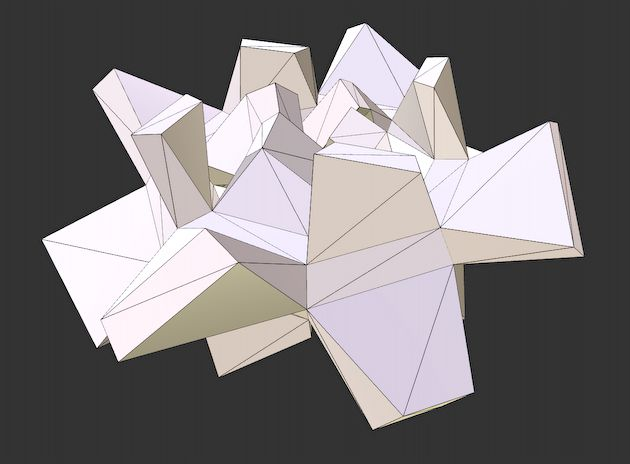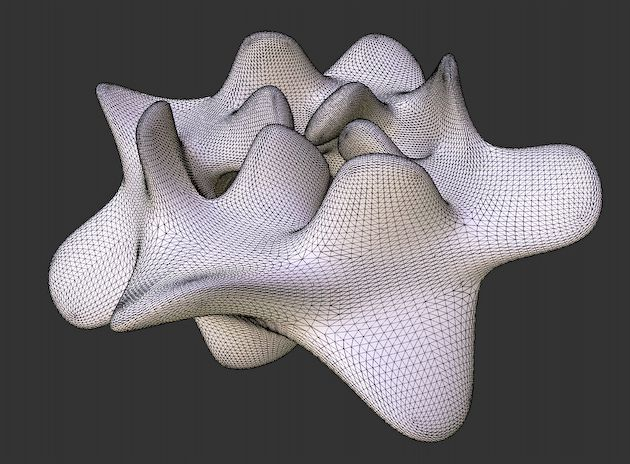```Mesh src_mesh;

Mesh subdiv_mesh;
MeshRefine::subdiv(subdiv_mesh, src_mesh, 4);
```

Conventionally, the process of creating LODs involves collapsing triangles throughout the entire model. This method works best for isolated objects, with the only drawback being LOD popping when the transition distance is too close to the camera. Only a single line is required to generate a simplified geometry from the high-polygonal model:

```Mesh reduce_mesh;
MeshReduce::collapse(reduce_mesh, subdiv_mesh, ratio);
```

The reduction ratio argument controls the number of primitives in the simplified mesh: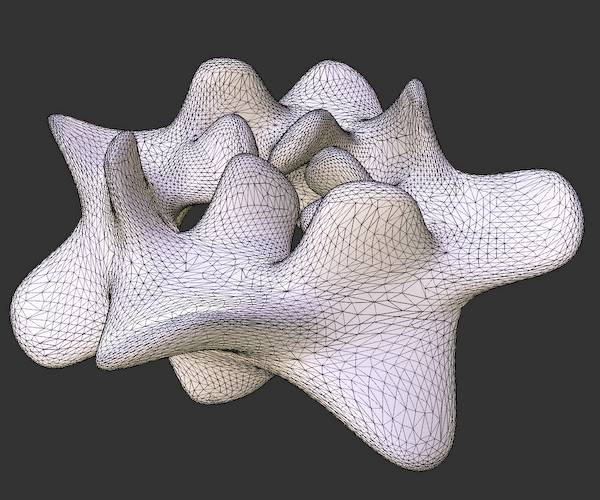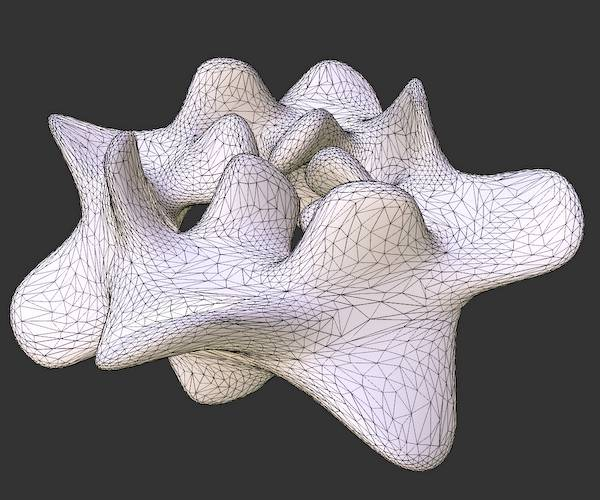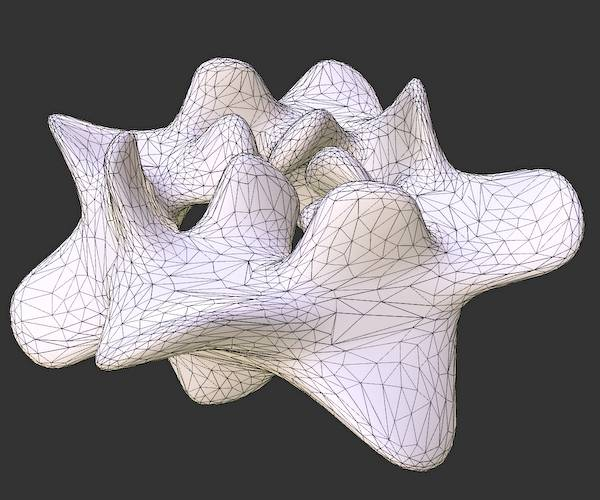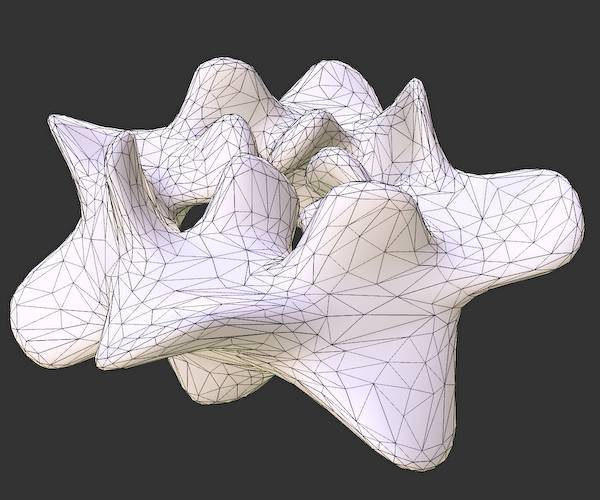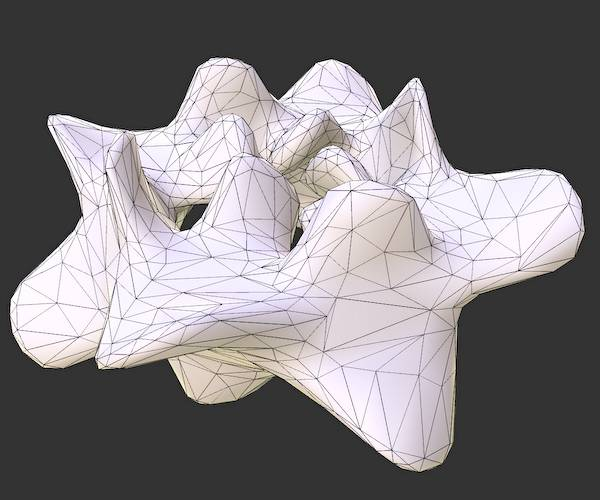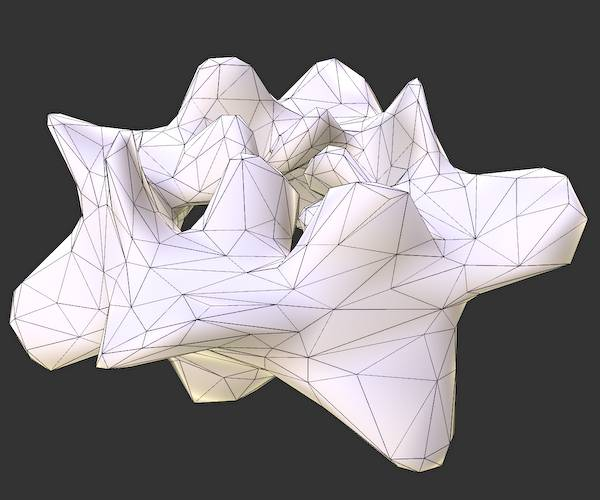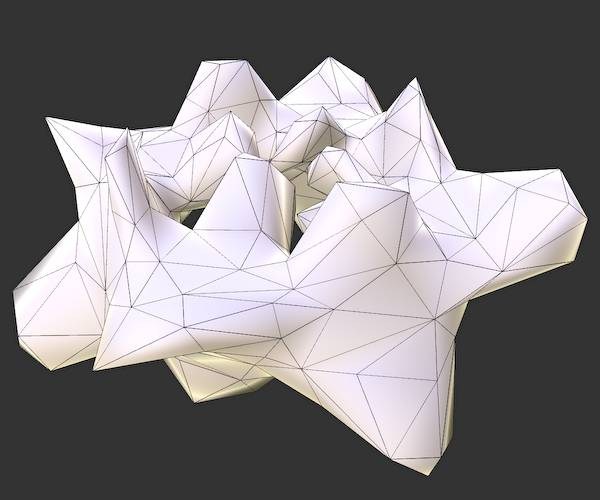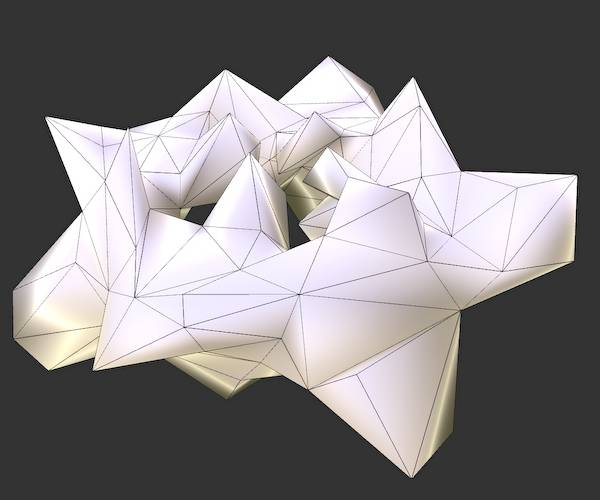After that, we can generate meshlets for each geometry and select it in the Task (Amplification) shader. Meshlets generation can be performed manually by calling Mesh::createIslands() method. Or the MeshModel interface will create them automatically by specifying MeshModel::FlagMeshlet during model creation. The main drawback of separated LODs is their inability to work with big objects where we need multiple LODs simultaneously. A spatial model splitting can be used as a workaround, but we will face cracks between splits with different LODs. But using Mesh shaders, we can choose a better solution involving hierarchical LOD graphs.

A LOD graph functions as a tree structure, wherein the root denotes the farthest LOD, and hierarchical children nodes represent distinct geometries. The distinguishing feature is that each node can have a second parent, transforming it into a graph rather than a conventional tree. You can find deeper insights about LOD graphs in the excellent Nanite SIGGRAPH 2021 paper. The generation of this LOG graph hierarchy is tricky, but the result is pretty straightforward. Tellusim Core SDK provides a MeshGraph::create() function where all the work is hidden inside. It’s possible to specify the maximum number of vertices and primitives per geometry to produce a perfect geometry split for different hardware and rendering algorithms. This function also supports multi-threaded generation for much faster processing times:

```Mesh graph_mesh;
MeshGraph::create(graph_mesh, subdiv_mesh, max_attributes, max_primitives);
```

And now, we can have different LODs in each model. With this approach, there are no cracks between levels, and the transition can be completely invisible if we choose the threshold that matches the screen pixel: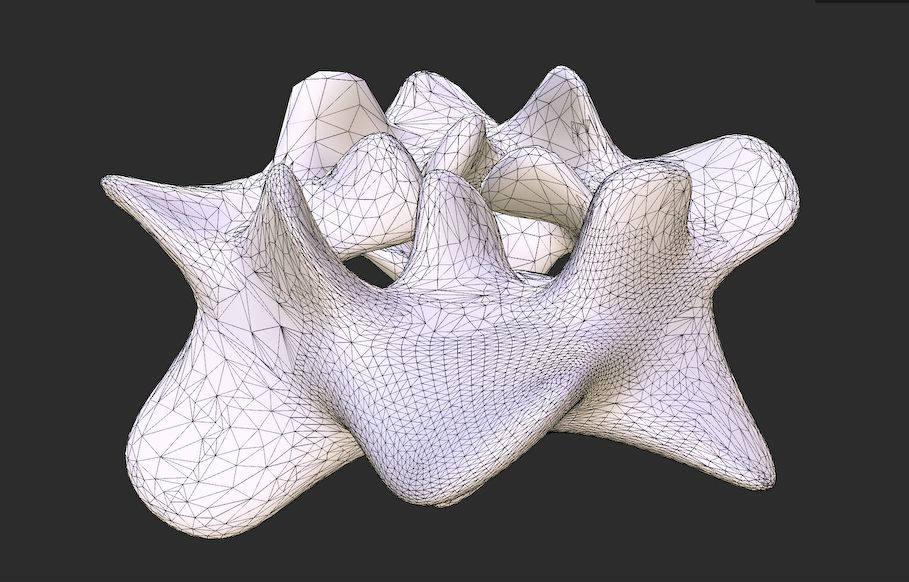Now we can return to our goal of drawing 8192 instances of this subdivided mesh. We will use a simple drawing algorithm with a single Task shader invocation per each instance. The Task shader will traverse a LOD graph and spawn Mesh shaders that draw our model. A multiple SSBO buffer will be used to make everything simple. The list of geometry hierarchy parameters that must be passed to the Task and Mesh shaders is described below:

```struct GeometryParameters {
Vector4f bound_min;             // bound box minimum
Vector4f bound_max;             // bound box maxumum
float32_t error;                // visibility error
uint32_t parent_0;              // first parent index
uint32_t parent_1;              // second parent index
uint32_t num_children;          // number of children geometries
uint32_t base_child;            // base child
uint32_t num_vertices;          // number of vertices
uint32_t base_vertex;           // base vertex
uint32_t num_indices;           // number of indices
uint32_t base_index;            // base index
};
```

Since we can manually specify the maximum number of vertices and indices per geometry, we can draw each geometry with a single Mesh shader invocation. The next snippet initializes geometry parameters:

```for(const MeshGeometry &geometry : mesh.getGeometries()) {

uint32_t index = geometry.getIndex();
GeometryParameters &parameters = geometry_parameters[index];

// bounding box
const BoundBoxf &bound_box = geometry.getBoundBox();
parameters.bound_min.set(bound_box.min, 1.0f);
parameters.bound_max.set(bound_box.max, 1.0f);

// visibility error
parameters.error = geometry.getVisibilityError();

// parent indices
parameters.parent_0 = (geometry.getParent0()) ? geometry.getParent0().getIndex() : Maxu32;
parameters.parent_1 = (geometry.getParent1()) ? geometry.getParent1().getIndex() : Maxu32;

// children indices
parameters.num_children = geometry.getNumChildren();
parameters.base_child = children_indices.size();
for(const MeshGeometry &child : geometry.getChildren()) {
children_indices.append(child.getIndex());
}

// vertices
parameters.num_vertices = min(mesh_model.getNumGeometryVertices(index), max_attributes);
parameters.base_vertex = mesh_model.getGeometryBaseVertex(index);

// indices
parameters.num_indices = min(mesh_model.getNumGeometryIndices(index), max_primitives * 3);
parameters.base_index = mesh_model.getGeometryBaseIndex(index);
}
```

After initializing all required buffers, the rendering function is simple:

```// set common parameters
command.setUniform(0, common_parameters);

// set storage buffers
command.setStorageBuffers(0, {
instances_buffer,
geometries_buffer,
children_buffer,
vertex_buffer,
index_buffer,
});

// draw instances
command.drawMesh(grid_size, grid_size, grid_height);
```

The Task shader performs parallel traversal of the LOD graph using a thread per geometry. A trick is to use two stacks: one for shared and one for local indices. This allows us to perform synchronization between threads in a minimal number of places, keeping most of the code parallel. We omit all visibility tests based on hierarchical Z for simplicity:

```void main() {

uint local_id = gl_LocalInvocationIndex;
uint group_id = (gl_WorkGroupID.z * grid_size + gl_WorkGroupID.y) * grid_size + gl_WorkGroupID.x;

[[branch]] if(local_id == 0u) {

// instance index
OUT.instance = group_id;

// instance transform
uint instance = group_id * 3u;
transform = instances_buffer[instance + 0u];
transform = instances_buffer[instance + 1u];
transform = instances_buffer[instance + 2u];

// clear geometries
num_geometries = 0;

// first geometry
shared_depth = 1;
shared_stack = 0;
}
memoryBarrierShared(); barrier();

// graph intersection
int local_depth = 0;
uint local_stack[LOCAL_STACK];

// stack barrier
memoryBarrierShared(); barrier();

// geometry index
int index = atomicDecrement(shared_depth) - 1;
[[branch]] if(index >= 0) {

// geometry index
uint geometry_index = shared_stack[index];

// transform bound box
vec3 bound_min = geometries_buffer[geometry_index].bound_min.xyz;
vec3 bound_max = geometries_buffer[geometry_index].bound_max.xyz;
transform_box(mat3x4(transform, transform, transform), bound_min, bound_max);

// check current geometry visibility
[[branch]] if(is_box_visible(bound_min, bound_max)) {

// geometry is visible
bool is_visible = true;

// distance to the bound box
float distance = get_box_distance(camera.xyz, bound_min, bound_max);

// the visibility error is larger than the threshold
[[branch]] if(distance < geometries_buffer[geometry_index].error * projection_scale) {

uint num_children = geometries_buffer[geometry_index].num_children;
uint base_child = geometries_buffer[geometry_index].base_child;

// draw geometry if this is a leaf
is_visible = (num_children == 0u);

// process children geometry
[[loop]] for(uint i = 0u; i < num_children; i++) {

// child geometry index
uint child_index = children_buffer[base_child + i];

// we came here from the second parent
[[branch]] if(geometries_buffer[child_index].parent_1 == geometry_index) {

// the first parent index
uint parent_index = geometries_buffer[child_index].parent_0;

// transform bound box
vec3 bound_min = geometries_buffer[parent_index].bound_min.xyz;
vec3 bound_max = geometries_buffer[parent_index].bound_max.xyz;
transform_box(mat3x4(transform, transform, transform), bound_min, bound_max);

// distance to the bound box
float distance = get_box_distance(camera.xyz, bound_min, bound_max);

// skip children if the first parent is visible
[[branch]] if(distance < geometries_buffer[parent_index].error * projection_scale) continue;
}

// next geometry to visit
[[branch]] if(local_depth < LOCAL_STACK) {
local_stack[local_depth++] = child_index;
}
}
}

// draw geometry
[[branch]] if(is_visible) {
int index = atomicIncrement(num_geometries);
[[branch]] if(index < MAX_GEOMETRIES) OUT.geometries[index] = geometry_index;
}
}
}

// minimal stack depth
atomicMax(shared_depth, 0);
memoryBarrierShared(); barrier();

// shared stack
[[loop]] for(int i = 0; i < local_depth && index < SHARED_STACK; i++) {
shared_stack[index++] = local_stack[i];
}
local_depth = 0;

// maximal stack depth
atomicMin(shared_depth, SHARED_STACK);
memoryBarrierShared(); barrier();
}

// emit meshes
}
```

The Mesh shader, in comparison to the Task shader, is trivial. We just load data from buffers and send it to the GPU. There is no vertex or index buffer compression to keep this example clear:

```void main() {

uint local_id = gl_LocalInvocationIndex;
uint group_id = gl_WorkGroupID.x;

// mesh parameters
[[branch]] if(local_id == 0u) {

// instance transformation
uint instance = IN.instance * 3u;
transform = instances_buffer[instance + 0u];
transform = instances_buffer[instance + 1u];
transform = instances_buffer[instance + 2u];

// geometry parameters
uint geometry = IN.geometries[group_id];
num_vertices = geometries_buffer[geometry].num_vertices;
num_primitives = geometries_buffer[geometry].num_indices / 3u;
base_vertex = geometries_buffer[geometry].base_vertex;
base_index = geometries_buffer[geometry].base_index;

// mesh color
float seed = mod(instance + geometry * 93.7351f, 1024.0f);
color = cos(vec3(0.0f, 0.5f, 1.0f) * 3.14f + seed) * 0.5f + 0.5f;
}
memoryBarrierShared(); barrier();

// number of primitives
SetMeshOutputsEXT(num_vertices, num_primitives);

// vertices
[[loop]] for(uint i = local_id; i < num_vertices; i += GROUP_SIZE) {

// fetch vertex
uint vertex = base_vertex + i;
vec4 position = vec4(vertices_buffer[vertex].position.xyz, 1.0f);
vec3 normal = vertices_buffer[vertex].normal.xyz;

// position
position = vec4(dot(transform, position), dot(transform, position), dot(transform, position), 1.0f);
gl_MeshVerticesEXT[i].gl_Position = projection * (modelview * position);

// camera direction
OUT[i].direction = camera.xyz - position.xyz;

// normal vector
OUT[i].normal = vec3(dot(transform.xyz, normal), dot(transform.xyz, normal), dot(transform.xyz, normal));

// color value
OUT[i].color = color;
}

// primitives
[[loop]] for(uint i = local_id; i < num_primitives; i += GROUP_SIZE) {

// fetch indices
uint index = base_index + i * 3u;
uint index_0 = indices_buffer[index + 0u];
uint index_1 = indices_buffer[index + 1u];
uint index_2 = indices_buffer[index + 2u];

// triangle indices
gl_PrimitiveTriangleIndicesEXT[i] = uvec3(index_0, index_1, index_2);
}
}
```

Without modification, this example can work with Vulkan, Direct3D12, or Metal API. There is no optimal number of Mesh shader vertices and primitives that will work best on all GPUs. But anyway, we can compare the performance of this app on different GPUs and APIs. An optimal LOD selection uses more than a single screen pixels threshold for better performance. The number of Task and Mesh shader threads per group is 32. The maximum number of vertices and primitives is set to 256:

Farthest LOD Optimal LOD Closest LOD
GeForce 3090 Ti 1400 FPS (0.6 ms) 300 FPS (3.4 ms) 15 FPS (64 ms)
GeForce 2080 Ti 1500 FPS (0.3 ms) 240 FPS (3.6 ms) 15 FPS (65 ms)
GeForce 3060 1100 FPS (0.7 ms) 115 FPS (8 ms) 6 FPS (166 ms)
Radeon 6900 XT 2200 FPS (0.3 ms) 350 FPS (2.3 ms) 22 FPS (43 ms)
Radeon 6700 XT 1300 FPS (0.5 ms) 210 FPS (4.1 ms) 11 FPS (86 ms)
Radeon 6600 1300 FPS (0.5 ms) 210 FPS (4.1 ms) 11 FPS (86 ms)
Intel Arc A770 540 FPS (1.7 ms) 110 FPS (8.9 ms) 5 FPS (205 ms)
Apple M1 Max 650 FPS 104 FPS N/A
Apple M1 215 FPS 25 FPS N/A

The difference between GPUs and modes is more important than absolute values. This scene configuration will be a reference point for future compute rasterization and ray tracing examples.